# Basic statistics in pandas DataFrame

Once you have cleaned your data, you probably want to run some basic statistics and calculations on your pandas DataFrame. It is really easy. Below I show some of the most common and basic statistics that you may want to use — there is a whole lot more to explore!

In the below examples, I am using a dataset I downloaded from Kaggle: Climate Change: Earth Surface Temperatures (https://www.kaggle.com/berkeleyearth/climate-change-earth-surface-temperature-data)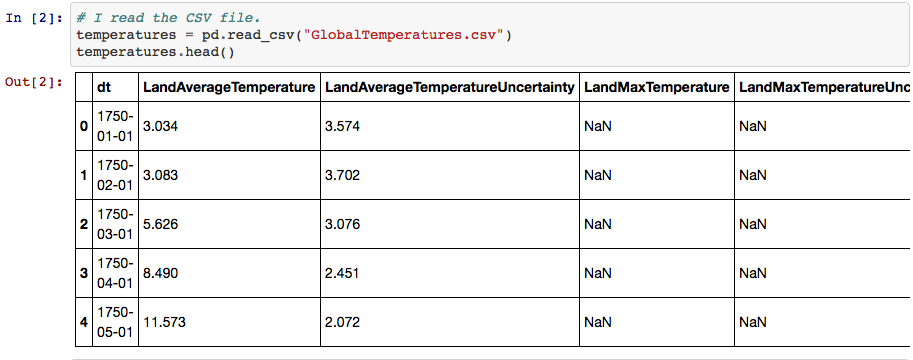The first five rows of my DataFrame

#### Sum

To add all of the values in a particular column of a DataFrame (or a Series), you can do the following:

df[‘column_name’].sum()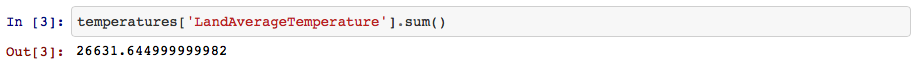Sum of all of the Land Average Temperatures

The above function skips the missing values by default. However, you can define that by passing a skipna argument with either True or False:

df[‘column_name’].sum(skipna=True)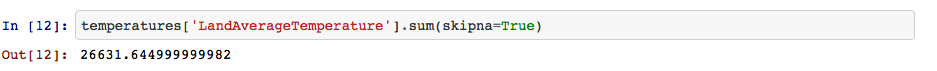You can see here that the sum is the same — because by default, the missing values are skipped

#### Arithmetic mean

df[‘column_name’].mean()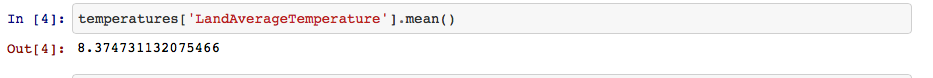Arithmetic mean for the Land Average Temperature

df.mean(axis=0)

Passing the argument of axis=0 returns the mean of every single column in the DataFrame:

df.mean(axis=1)

Passing the argument of axis=1 will return the mean of every single row in the DataFrame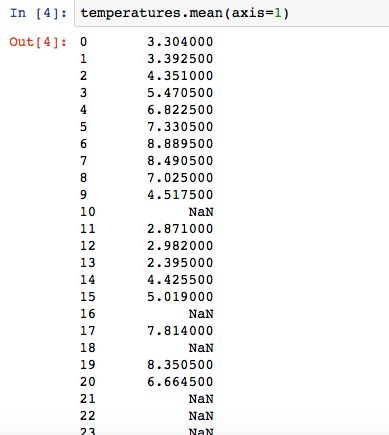Mean of each row in the temperatures DataFrame

#### Summary statistics

df[‘column_name’].describe()

This function gives you several useful things all at the same time. For example, you will get the three quartiles, mean, count, minimum and maximum values and the standard deviation. This is very useful, especially in exploratory data analysis.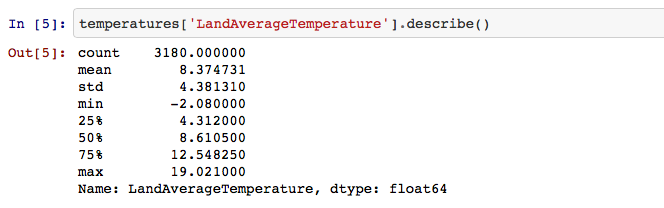A bunch of different stats for the Land Average Temperature

df[‘column_name’].describe(percentiles=[percentile1, percentile2, percentile3, percentile4]

You can also choose specific percentiles to be included in the describe method output by adding the percentiles argument and specifying. You can change the number of percentiles you ask for as you please — 4 percentiles are just an example.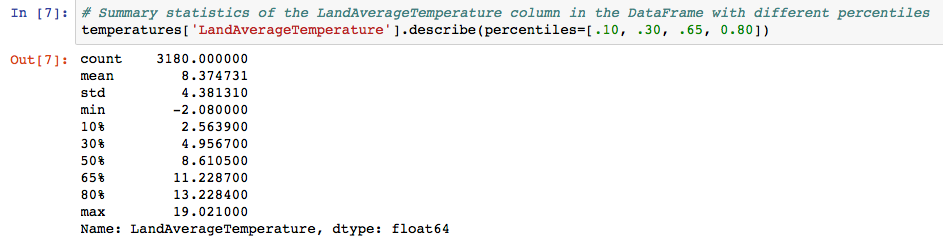Summary statistics with four odd percentiles

Note: If your object is non-numerical, the summary statistics will be sligthly different. They will include the count, frequency, the number of unique values and the top value.
If your object contains both numerical and non-numerical values, the describe method will only include summary statistics of the numerical values.

#### Counting the number of values

df[‘column_name’].count()

Here, you will get the number of values you have in the column.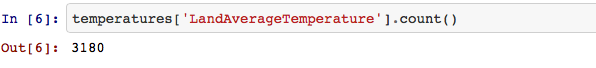You can also see the same number above, when I used ‘describe’

#### Maximum and minimum value

df[‘column_name’].max()

Finding the maximum value from a column of a DataFrame or a Series. You probably get the idea by now.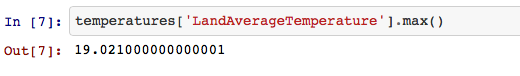The maximum temperature in the Land Average Temperature

df[‘column_name’].min()

Finding the minimum value from the column of a DataFrame or a Series.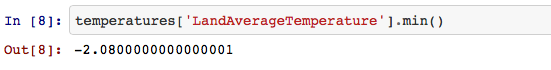The minimum temperature in the Land Average Temperature

#### Median

df[‘column_name’].median()

Finding the median: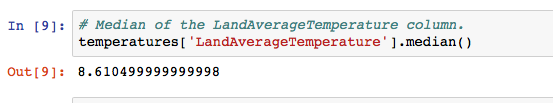Median of the LandAverageTemperature column.

#### Mode

df[‘column_name’].mode()

Finding the mode: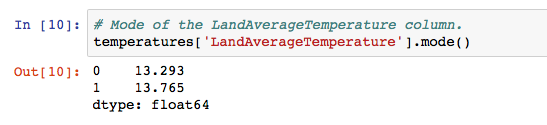Mode of the Land Average Temperature

Of course, there are a lot of other statistics you may need to use — rolling mean, variance or standard deviation to mention just a few.

All the code can be found on GitHub: https://github.com/kasiarachuta/Blog/blob/master/Basic%20statistics%20on%20pandas%20DataFrame.ipynb